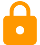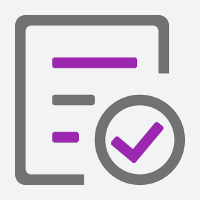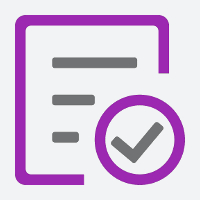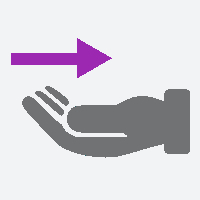Select Page

# Elementary-level EFL - ESL lesson plans | A2

A collection of ESL lesson plans and resources for teaching elementary-level students. All of the activity and game ideas that we include can be used in low-resource classrooms and require little to no preparation time. Each lesson contains a warm-up activity with board work, several classroom activities, games and a collection of recommended free ESL resources from around the web. If you prefer to work with paper, there are printable versions of each lesson available to members of the site. You can check out a sample here.LESSON 1
Question words
This ESL lesson plan gives students a chance to practise all of the common question structures.
A2+LESSON 2
Countable and uncountable nouns
In this elementary-level lesson plan, students will expand on their food-related vocabulary while deepening their knowledge of countable and uncountable nouns.
A2+LESSON 3
Expressing quantities
This ESL lesson plan covers some of the more common quantifiers used to express large and small quantities.
A2+LESSON 4
Using the present simple
An ESL lesson plan that focuses on the functional uses of the present simple.
A2+LESSON 5
Using the present continuous
A collection of teaching resources that can be used to deepen a student's understanding of the present continuous.A2+LESSON 6
Relative pronouns
This ESL lesson plan contains activities and games that will help elementary level students familiarise themselves with relative pronouns and their function in the English language.A2+LESSON 7
Personal pronouns
Students learn to work with personal pronouns in this elementary-level ESL lesson plan.
A2+LESSON 8
Comparatives and superlatives
This elementary-level lesson plan focuses on the correct use of comparative and superlative adjectives.A2+LESSON 9
Using the past simple
This ESL lesson plan teaches students about the functional uses of the past simple.A2+LESSON 10
Past simple and past continuous
In this grammar lesson, students learn how to combine the present simple and continuous tenses.
A2+
Cut down on planning. Teach with confidence.
Try the TEFL Handbook mobile app. Perfect for quick reference in the classroom.LESSON 11
An elementary-level lesson plan that teaches students how to express the future using 'going to + Infinitive' and 'will'.A2+LESSON 12
The present perfect
In this ESL lesson plan, students will learn the grammar and function of the present perfect tense.
A2+LESSON 13
The present perfect: Since and for
This ESL lesson plan builds on the previous lesson's material and teaches students how to use 'since' and 'for' with the present perfect.
A2+LESSON 14
The present perfect: Just, yet and already
For this lesson, students will learn how to use the present perfect with the time modifiers 'just', 'yet' and 'already'.A2+LESSON 15
This ESL lesson plan contains activities and games that teach elementary-level students how to express obligation, prohibition and advice.A2+LESSON 16
Expressing possibility: May and might
An ESL lesson plan that teaches elementary level students how to express possibility using 'may' and 'might'.
A2+LESSON 17
Necessity
Students learn how to express necessity through these engaging ESL activities and games.
A2+LESSON 18
Verb patterns
In this grammar lesson plan, students learn how to use some common verb patterns.A2+LESSON 19
The first conditional
ESL activities, games and resources for teaching the first conditional to elementary level students.A2+LESSON 20
Indefinite pronouns
This lesson plan contains a fun selection of ESL activities and games that can be used by elementary level students to practise using indefinite pronouns.
A2+
Plan your lessons on the go
A mobile app for English teachers